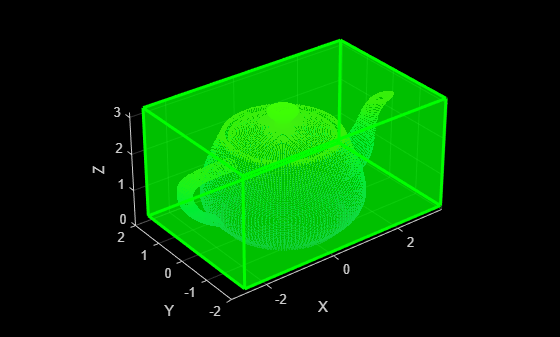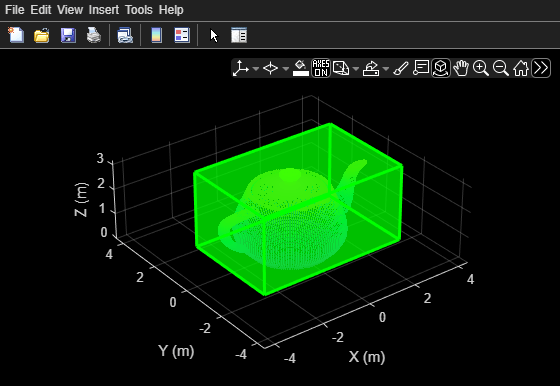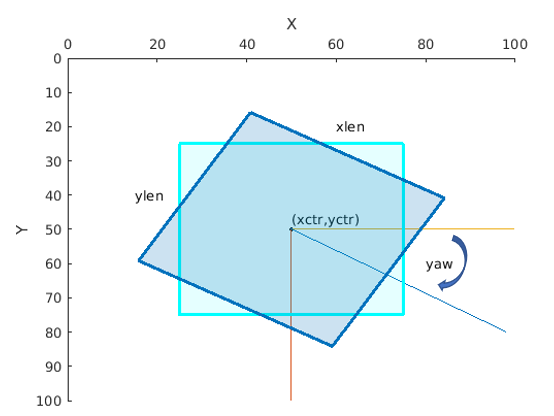# showShape

Display shapes on image, video, or point cloud

## Syntax

``showShape(shape,position)``
``showShape(___,Name,Value)``

## Description

example

````showShape(shape,position)` displays one or more instances of a shape `shape` in the current axes at the specified locations `position`.```

example

````showShape(___,Name,Value)` specifies options using one or more name-value pair arguments. For example, '`Color'`,`'yellow`' specifies the color of the displayed shapes as yellow.```

## Examples

collapse all

Read an image into the workspace.

`I = imread('visionteam1.jpg');`

Create an aggregate channel features (ACF) people detector.

`detector = peopleDetectorACF()`
```detector = acfObjectDetector with properties: ModelName: 'inria-100x41' ObjectTrainingSize: [100 41] NumWeakLearners: 2048 ```

Detect people in the image.

`[bboxes,scores] = detect(detector,I);`

Display the image with a labeled rectangle, including the associated detection score, around each detected person.

```figure imshow(I) labels = "person:"+scores; showShape('rectangle',bboxes,'Label',labels)```Read point cloud data into the workspace.

`ptCloud = pcread('teapot.ply');`

Display the point cloud data.

```figure pcshow(ptCloud) xlabel('X') ylabel('Y') zlabel('Z')```

Define a cuboid and display it in green with an opacity of `0.5`.

```pos = [0.3753 0 1.65 6 4 3 0 0 0]; showShape('cuboid',pos,'Color','green','Opacity',0.5)```Read point cloud data into the workspace.

`ptCloud = pcread('teapot.ply');`

Define a rotation matrix and 3-D transform to rotate the point cloud, and an associated cuboid, by 5 degrees.

```rot = 5; R = [ cosd(rot) sind(rot) 0 0; ... -sind(rot) cosd(rot) 0 0; ... 0 0 1 0; ... 0 0 0 1]; tform = affine3d(R);```

Compute the x and y rendered limits of the point cloud to ensure that the point cloud is not clipped during rotation.

```pcLimits = abs([ptCloud.XLimits ptCloud.YLimits]); maxLimit = max(pcLimits);```

Add an additional margin to the plot to prevent the cuboid from being clipped during rotation.

```margin = 1; maxLimit = maxLimit + margin; xlimits = [-maxLimit maxLimit]; ylimits = [-maxLimit maxLimit]; zlimits = ptCloud.ZLimits;```

Create a player for visualizing the point cloud.

`player = pcplayer(xlimits,ylimits,zlimits);`

Customize the player axis labels.

```xlabel(player.Axes,'X (m)'); ylabel(player.Axes,'Y (m)'); zlabel(player.Axes,'Z (m)');```

Define a cuboid around the point cloud.

`cuboidPosition = [0.3753 0 1.65 6 4 3 0 0 0];`

Define the output view for a cuboid rotation. Use the same limits as the player so that the cuboid is not clipped. Then, display the rotated point cloud and cuboid.

```gridSize = [1 1 1]; ref = imref3d(gridSize,xlimits,ylimits,zlimits); for i = 1:round((360/rot)) % Rotate point cloud. ptCloud = pctransform(ptCloud,tform); % Rotate the cuboid. cuboidPosition = bboxwarp(cuboidPosition,tform,ref); % Show rotated point cloud data. view(player,ptCloud) % Show rotated cuboid. showShape('cuboid',cuboidPosition, ... 'Parent',player.Axes, ... 'Color','green', ... 'Opacity',0.5) % Use drawnow to synchronize point cloud and shape visualization. drawnow end```## Input Arguments

collapse all

Type of shape, specified as a character vector. The vector can be `'line'`, `'rectangle'`, `'cuboid`, `'polygon'`, or `'circle'`.

Data Types: `string`

Positions and sizes of shapes, specified according to the type of shapes, described in this table.

Bounding BoxDescription
Axis-aligned rectangle

Defined in pixel coordinates as an M-by-4 numeric matrix with rows of the form [x y w h], where:

• M is the number of axis-aligned rectangles.

• x and `y` specify the upper-left corner of the rectangle.

• w specifies the width of the rectangle, which is its length along the x-axis.

• h specifies the height of the rectangle, which is its length along the y-axis.

Rotated rectangle

Defined in spatial coordinates as an M-by-5 numeric matrix with rows of the form [xctr yctr xlen ylen yaw], where:

• M is the number of rotated rectangles.

• xctr and yctr specify the center of the rectangle.

• xlen specifies the width of the rectangle, which is its length along the x-axis before rotation.

• ylen specifies the height of the rectangle, which is its length along the y-axis before rotation.

• yaw specifies the rotation angle in degrees. The rotation is clockwise-positive around the center of the bounding box.Cuboid

Defined in spatial coordinates as an M-by-9 numeric matrix with rows of the form [xctr yctr zctr xlen ylen zlen xrot yrot zrot], where:

• M is the number of cuboids.

• xctr, yctr, and zctr specify the center of the cuboid.

• xlen, ylen, and zlen specify the length of the cuboid along the x-axis, y-axis, and z-axis, respectively, before rotation.

• xrot, yrot, and zrot specify the rotation angles of the cuboid around the x-axis, y-axis, and z-axis, respectively. The xrot, yrot, and zrot rotation angles are in degrees about the cuboid center. Each rotation is clockwise-positive with respect to the positive direction of the associated spatial axis. The function computes rotation matrices assuming `ZYX` order Euler angles [xrot yrot zrot].

The figure shows how these values determine the position of a cuboid.`Circle`

Defined in spatial coordinates as an M-by-3 numeric matrix with rows of the form [xctr yctr radius], where:

• M is the number of circles.

• xctr and yctr specify the center of the circle.

`Line`

Defined in spatial coordinates as a P-by-2 matrix in which each row is an [x y] endpoint or a 1-by-2P vector of consecutive endpoints of the from [x1 y1 x2 y2xP xP].

• P is the number of endpoints.

• [x1 y1], [x2 y2], and xP xP describe endpoints.

To specify several lines that contain different numbers of endpoints, you can use the cell array format described for a polygon.

`Polygon`

Defined in spatial coordinates as an M-by-1 cell array in which each cell contains an L-by-2 matrix of [x y] vertex locations or a 1-by-2L vector of consecutive vertex locations of the form [x1, y1, x2,y2, … xL,yL ], where:

• M is the number of polygons.

• [x1 y1], [x2 y2] describe vertex locations or endpoints.

• L is the number of vertices in a polygon or endpoints in a line. Each polygon or line can have different number of vertices or endpoints.

### Name-Value Pair Arguments

Specify optional comma-separated pairs of `Name,Value` arguments. `Name` is the argument name and `Value` is the corresponding value. `Name` must appear inside quotes. You can specify several name and value pair arguments in any order as `Name1,Value1,...,NameN,ValueN`.

Example: '`Color'`,`'yellow`' specifies the color of the displayed shapes as yellow.

Shape label, specified as the comma-separated pair consisting of `'Label'` and either a scalar, M-element vector, or an M-element cell array of character vectors.

If the input is a scalar value, then the function applies that same value as a label to each shape. If the input is an M-element vector or cell array, then the function applies each value to the corresponding shape, using the order in which the shapes are specified to the function. M is the number of shapes specified to the function.

Shape color, specified as the comma-separated pair consisting of `'Color'` and one or more RGB triplets, or one or more color names or short color names. To use the same color for all shapes, specify a single (MATLAB `ColorSpec`) color name or a single RGB triplet.

To use a different color for each shape, specify an M-element vector or cell array of color names or an M-by-3 numeric matrix in which each row is an RGB triplet. M is the number of shapes specified to the function.

Opacity of the shape fill, specified as the comma-separated pair consisting of `'Opacity'` and an M-element vector of numeric values in the range [0 1]. To use the same opacity for all shapes, specify a scalar opacity value. For a fully opaque shape fill, set `'Opacity'` to `1`.

Output axes, specified as the comma-separated pair consisting of `'Parent'` and an Axes Properties graphics object.

Border line color, specified as the comma-separated pair consisting of `'Color'` and one or more RGB triplets, or one or more color names or short color names. To use the same color for all border lines, specify a single (MATLAB `ColorSpec`) color name or a single RGB triplet.

To use a different color for each shape, specify an M-element vector or cell array of color names or an M-by-3 numeric matrix in which each row is an RGB triplet. M is the number of shapes specified to the function.

Border line width in pixels, specified as the comma-separated pair consisting of `'LineWidth'` and a positive scalar integer or an M-element vector of positive scalar integers. To use the same line width for all shapes, specify a positive scalar integer. Otherwise, specify an M-element vector of positive integers, where M is the number of shapes specified to the function.

Border line opacity of the shape fill, specified as the comma-separated pair consisting of `'Opacity'` and an M-element vector of numeric values in the range [0 1]. To use the same opacity for the border line of all shapes, specify a scalar opacity value. For a fully opaque border line, set `'Opacity'` to `1`.

Label text color, specified as the comma-separated pair consisting of `'Color'` and one or more RGB triplets, or one or more color names or short color names. To use the same color for the text of all labels, specify a single (MATLAB `ColorSpec`) color name or a single RGB triplet.

To use a different color for text of each label, specify an M-element vector or cell array of color names or an M-by-3 numeric matrix in which each row is an RGB triplet. M is the number of labels specified to the function.

Label opacity, specified as the comma-separated pair consisting of `'Opacity'` and an M-element vector of numeric values in the range [0 1]. To use the same opacity for all labels, specify a scalar opacity value. For a fully opaque label, set `'Opacity'` to `1`.

Label font, specified as the comma-separated pair consisting of `'LabelFont'` and `'FixedWidth'` or a font that your system supports. To display and print text properly, you must select a font that your system supports. If you select an unsupported font, the function returns an error. If you specify `'FixedWidth'`, the function uses the font specified by the `FixedWidthFont` property of the Root Properties object. The fixed-width font relies on the value of `get(0,'FixedWidthFontName')` .

Label font size in point units, specified as the comma-separated pair consisting of `'LabelFontSize'` and a positive scalar.